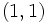# Subgroup structure of dihedral group:D16

Jump to: navigation, search
This article gives specific information, namely, subgroup structure, about a particular group, namely: dihedral group:D16.
View subgroup structure of particular groups | View other specific information about dihedral group:D16

This article discusses the subgroup structure of dihedral group:D16, the dihedral group of order sixteen (and hence, degree eight). We use here the presentation:$G := \langle a,x \mid a^8 = x^2 = e, xax = a^{-1} \rangle$.$G$ has 16 elements:$\{ e, a, a^2, a^3, a^4, a^5, a^6, a^7, x, ax, a^2x, a^3x, a^4x, a^5x, a^6x, a^7x \}$

## Tables for quick information

FACTS TO CHECK AGAINST FOR SUBGROUP STRUCTURE: (group of prime power order)
Lagrange's theorem (order of subgroup times index of subgroup equals order of whole group, so all subgroups have prime power orders)|order of quotient group divides order of group (and equals index of corresponding normal subgroup, so all quotients have prime power orders)
prime power order implies not centerless | prime power order implies nilpotent | prime power order implies center is normality-large
size of conjugacy class of subgroups divides index of center
congruence condition on number of subgroups of given prime power order: The total number of subgroups of any fixed prime power order is congruent to 1 mod the prime.

### Table classifying subgroups up to automorphisms

In case a single equivalence class of subgroups under automorphisms comprises multiple conjugacy classes of subgroups, outer curly braces are used to bucket the conjugacy classes.

Automorphism class of subgroups List of subgroups Isomorphism class Order of subgroups Index of subgroups Number of conjugacy classes (=1 iff automorph-conjugate subgroup) Size of each conjugacy class (=1 iff normal subgroup) Total number of subgroups (=1 iff characteristic subgroup) Isomorphism class of quotient (if exists) Subnormal depth Nilpotency class
trivial subgroup$\{ e \}$ trivial group 1 16 1 1 1 dihedral group:D16 1 0
center of dihedral group:D16$\{ e, a^4 \}$ cyclic group:Z2 2 8 1 1 1 dihedral group:D8 1 1
non-normal order two subgroups of dihedral group:D16$\{ \{ e,x \}, \{ e, a^2x \}, \{ e, a^4x \}, \{ e, a^6x \} \}$$\{ \{ e, ax \}, \{ e, a^3x \}, \{ e, a^5x \}, \{ e, a^7x \} \}$
cyclic group:Z2 2 8 2 4 8 -- 3 1
derived subgroup of dihedral group:D16$\{ e, a^2, a^4, a^6 \}$ cyclic group:Z4 4 4 1 1 1 Klein four-group 1 1
Klein four-subgroups of dihedral group:D16$\{ \{ e, a^4, x, a^4x \}, \{ e, a^4, a^2x, a^6x \} \}$$\{ \{ e, a^4, ax, a^5x \}, \{ e, a^4, a^3x, a^7x \} \}$
Klein four-group 4 4 2 2 4 -- 2 1
Cyclic maximal subgroup of dihedral group:D16$\{ e, a, a^2, a^3, a^4, a^5, a^6, a^7 \}$ cyclic group:Z8 8 2 1 1 1 cyclic group:Z2 1 1
D8 in D16$\langle a^2, x \rangle$,$\langle a^2, ax \rangle$ dihedral group:D8 8 2 2 1 2 cyclic group:Z2 1 2
whole group all elements dihedral group:D16 16 1 1 1 1 trivial group 0 3
Total (8 rows) -- -- -- -- 11 -- 19 -- -- --

### Table classifying isomorphism types of subgroups

The first part of the GAP ID is the order of the subgroup.

Group name GAP ID Index Occurrences as subgroup (=1 iff isomorph-free subgroup) Conjugacy classes of occurrence as subgroup (=1 iff isomorph-conjugate subgroup) Automorphism classes of occurrence as subgroup (=1 iff isomorph-automorphic subgroup) Occurrences as normal subgroup (=1 iff normal-isomorph-free subgroup; =Occurrences as subgroup iff isomorph-normal subgroup) Occurrences as characteristic subgroup (=1 iff characteristic-isomorph-free subgroup; =Occurrences as subgroup iff isomorph-characteristic subgroup)
Trivial group$(1,1)$ 16 1 1 1 1 1
Cyclic group:Z2$(2,1)$ 8 9 3 2 1 1
Cyclic group:Z4$(4,1)$ 4 1 1 1 1 1
Klein four-group$(4,2)$ 4 4 2 1 0 0
Cyclic group:Z8$(8,1)$ 2 1 1 1 1 1
Dihedral group:D8$(8,3)$ 2 2 2 1 2 0
Dihedral group:D16$(16,7)$ 1 1 1 1 1 1
Total (7 rows) -- -- 19 11 8 7 5

### Table listing number of subgroups by order

Note that due to the congruence condition on number of subgroups of given prime power order, the number of subgroups of any fixed order is congruent to 1 mod 2, i.e., is odd.

Group order Index Occurrences as subgroup Conjugacy classes of occurrence as subgroup Automorphism classes of occurrence as subgroup Occurrences as normal subgroup Occurrences as characteristic subgroup
1 16 1 1 1 1 1
2 8 9 3 2 1 1
4 4 5 3 2 3 1
8 2 3 3 2 1 1
16 1 1 1 1 1 1
Total -- 19 11 8 7 5